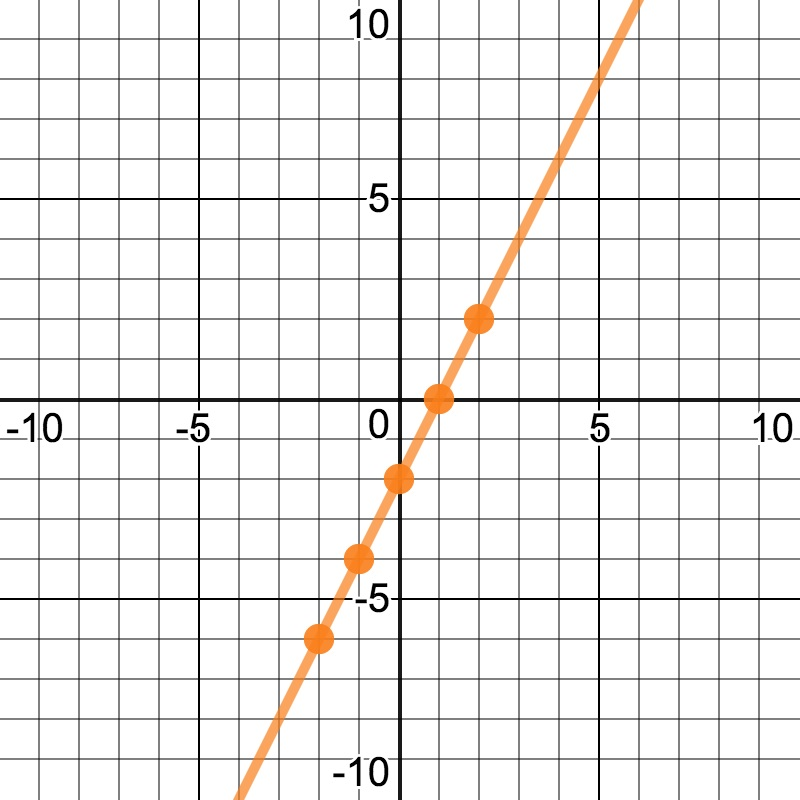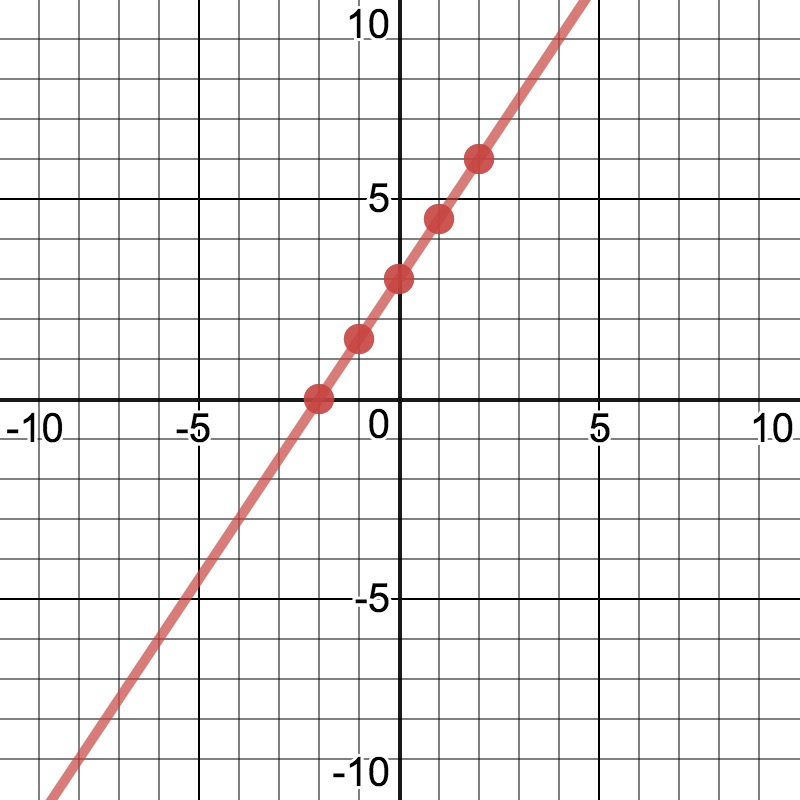# POLQ 3 | Lesson 1 | Practice (Computation of Functions)# Computation of Functions

You are given the functions below. You will use these functions to create new functions, much as you did in the Explore. The directions are below the four functions.

$$f(x) = \dfrac{1}{2}(x+3)$$$$g(x) = 2(x-1)$$;$$h(x) = \dfrac{3}{2}(x+2)$$;$$j(x) = x^2$$.On graph paper or using Desmos, used what you learned in the first part of this activity to graph the function computations below the use several points on each graph to create a new graphs. Are there any patterns in the resulting graphs?

1) $$f(x)+g(x)$$

2) $$f(x)+h(x)$$

3) $$f(x)+j(x)$$

4) $$f(x)-g(x)$$

5) $$f(x) \cdot h(x)$$

6) $$f(x) \cdot g(x) \cdot h(x)$$